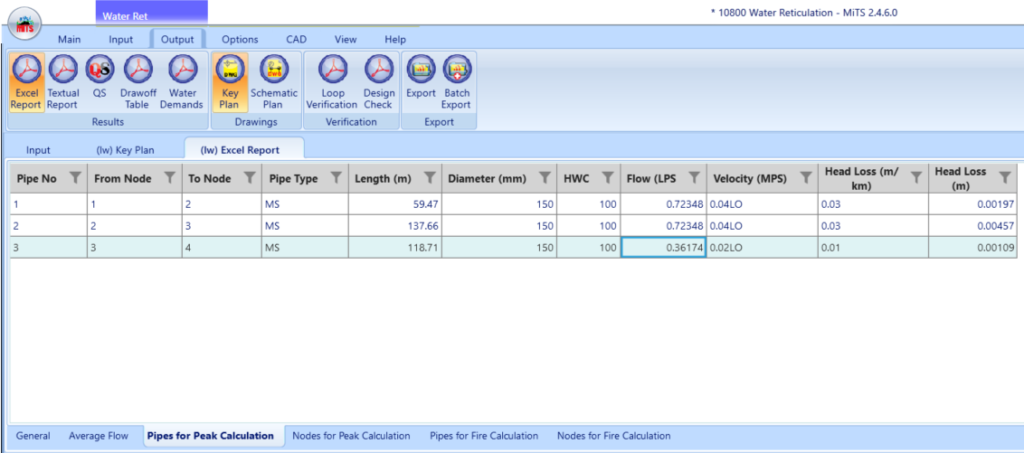603 - 5885 1250 (4 lines) info@mes100.com
Select Page

Water reticulation is water distribution network which is delivered to the consumers. The distribution came from the pressure created from the source and delivered to its destination. Below is an example of the calculation and benchmark with MiTS software.

Provided a project file and the spreadsheet as your reference.

Diagram below displays the common terms used in water reticulation.

Hydraulic Grade Line (HGL)

Residual Pressure

= Hydraulic Grade Line – Highest Supply Level

Tank Water Level

=  Height from Ground Level to Tank Water Level

Highest Supply Level

= Ground Level + Tank Water Level

= Using Hazen William equation
(Manual Headloss Calculation Benchmark)

# Peak Flow

Peak flow or also known as domestic flow. It is calculated for water distribution for domestic uses (residential, school, commercial etc.). Water demand is multiplied with peak factor (2.5) to determine the flow.

i. Example on Pipe 3 in Nodes for Peak Calculation

Flow (LPS) = Water Demand x Peak Factor

= |--0.144696211458333| x 2.5

= 0.3617 LPSCalculation of Flow in Pipes for Peak Calculation in MiTS

Velocity (m/s) = Q/A

= $\frac { \frac { 0.3617 }{ 1000 } }{ { \Pi 0.075 }^{ 2 } }$

= 0.0205 m/s

Energy Slope (m/km) = ${ \sqrt [ 27 ]{ \frac { V }{ k\quad C\quad { (\frac { D }{ 4 } ) }^{ 0.63 } } } }^{ 50 }$ x 1000

(you may refer here)

= ${ \sqrt [ 27 ]{ \frac { 0.0204703 }{ 0.85\quad x\quad 100\quad x\quad (\frac { 0.15 }{ 4 } { ) }^{ 0.63 } } } }^{ 50 }$

= 0.009185 m/km

Head Loss (m) = Sf x L

= $\frac { 0.009185x118.7105 }{ 1000 }$

= 0.00109 m

ii. Example on Pipe 1 in Pipes for Peak Calculation

HGL = Fix Head – Head Loss

= 60 – 0.002

= 59.998 m

HSL = Ground Level + Tank Water Level

= 33.22 + 0

= 33.22 m

Residual Pressure = HGL – HSL

= 59.998 – 33.22

= 26.778 m

# Fire Calculation

Fire calculation is the calculation with hydrant assigned in the design. The purpose of it is to make sure whether the water distribution is sufficient to cater during fire breakout, even at the lowest pressure. The value usually used for water demand in fire calculation is 22.7 LPS.

i. Example on Pipe 3 in Nodes for Fire Calculation

Flow (LPS) = (Water Demand x Fire Factor) + Average Flow

= (22.7 x 1) + 0.145

= 22.845 LPS

Velocity (m/s) = Q/A

= $\frac { \frac { 22.84 }{ 1000 } }{ \Pi { 0.075 }^{ 2 } }$

= 1.292 m/s

Energy Slope (m/km) = ${ \sqrt [ 27 ]{ \frac { V }{ kC{ (\frac { D }{ 4 } ) }^{ 0.63 } } } }^{ 50 }$

= ${ \sqrt [ 27 ]{ \frac { 1.292 }{ 0.85x100x{ (\frac { 0.15 }{ 4 } ) }^{ 0.63 } } } }^{ 50 }$ x 1000

= 19.80 m/km

Head Loss (m) = Sf x L

= 19.80 x 118.71 / 1000

= 2.35 m

ii. Example on Pipe 1 in Pipes for Fire Calculation

HGL = Fix Head – Head Loss

= 60 – 1.19

= 58.81 m